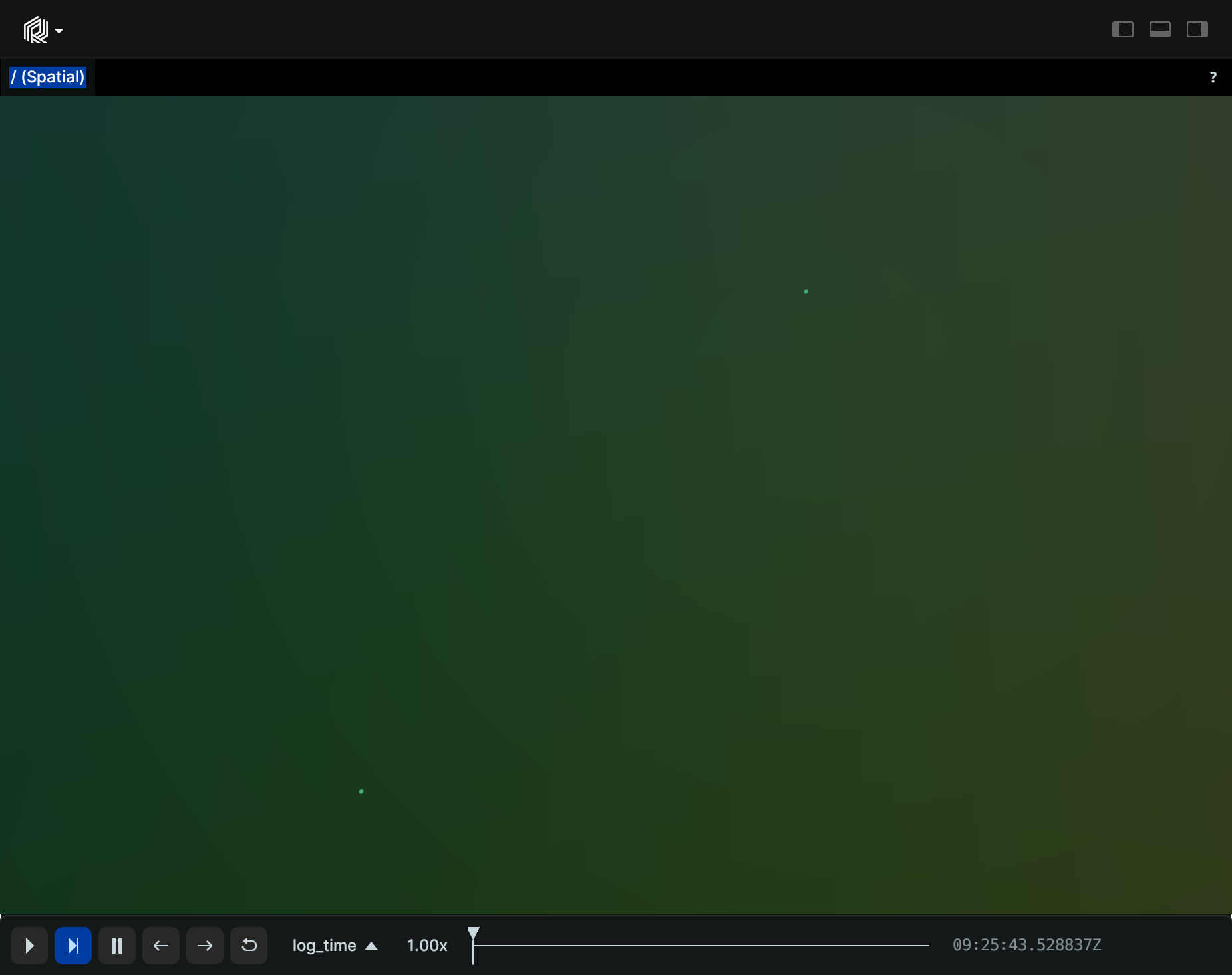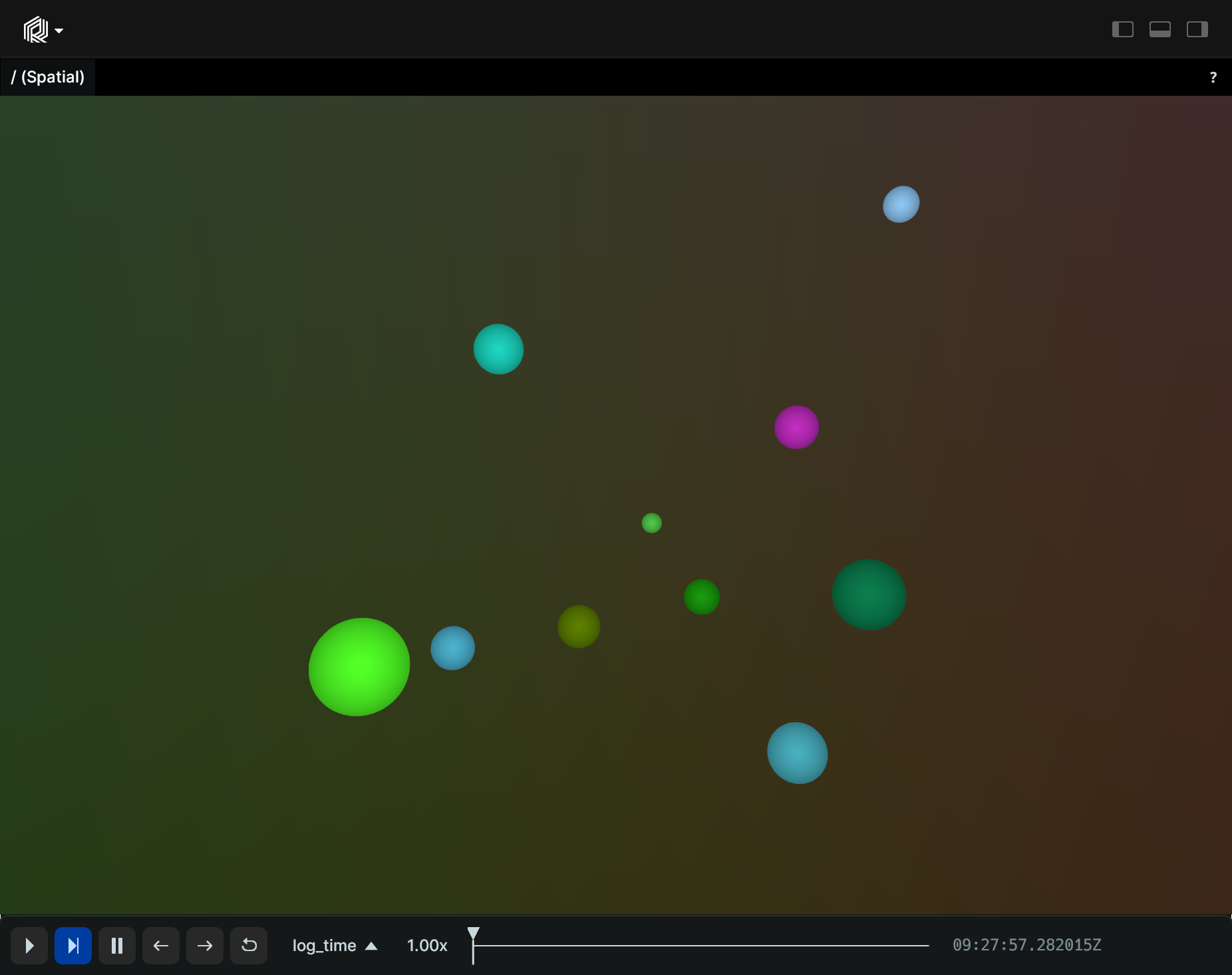# Points3D

A 3D point cloud with positions and optional colors, radii, labels, etc.

## Components

Required: `Position3D`

Recommended: `Radius`, `Color`

## Examples

### Simple 3D points

``````"""Log some very simple points."""
import rerun as rr

rr.init("rerun_example_points3d_simple", spawn=True)

rr.log("points", rr.Points3D([[0, 0, 0], [1, 1, 1]]))
``````### Randomly distributed 3D points with varying color and radius

``````"""Log some random points with color and radii."""
import rerun as rr
from numpy.random import default_rng

rr.init("rerun_example_points3d_random", spawn=True)
rng = default_rng(12345)

positions = rng.uniform(-5, 5, size=[10, 3])
colors = rng.uniform(0, 255, size=[10, 3])